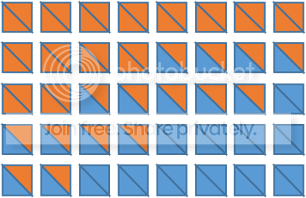# How to best display results from a factorial design?

How can I create plots in R like this mockup made in Powerpoint?In other words, for each combination of x-axis and y-axis values, I want to plot two triangles which, together, form a square. I have two vectors of values, one of which determines the color of the lower triangle, and the other of which determines the color of the upper triangle. I can't figure out how to plot triangles which will fit together the way I want.

Here's sample code:

# generate sample data
D = data.frame( expand.grid( x=seq(1,10,1), y=seq(1,10,1) ) )
D$color1 = sample( c('red','blue'), nrow(D), replace=TRUE ) D$color2 = sample( c('red','blue'), nrow(D), replace=TRUE )

# this will plot just one color in each cell, not two as I want:
plot( D$x, D$y, col=D$color1, pch=15, cex=4 )  In case it matters - the point of the plot is to show the results of simulated experiments for each combination of values of parameters x and y. Thus, each box represents the outcome of one simulation. It's a 2x2 experimental design with factors I'll call A and B, each with 2 levels, A1/A2 and B1/B2. So red color means A1>A2 on the outcome measure, and blue means A2>A1. Above the diagonal represents the result for B=B1 and below the diagonal represents the result for B=B2. Note, by the way, that I need to make several such plots, one for each value of a third parameter I'll call z. I'm open to suggestions if someone thinks there is a better way to visualize this data. ## 1 Answer Here is one solution that uses the my.symbols function from the TeachingDemos package: library(TeachingDemos) ms.tripair <- function(c1,c2) { polygon( c(-1,-1,1,-1), c(-1,1,-1,-1), col=c1 ) polygon( c(-1,1,1,-1), c(1,1,-1,1), col=c2 ) } my.symbols( D$x, D$y, ms.tripair, c1=D$color1, c2=D\$color2,
add=FALSE, symb.plots=TRUE, inches=0.4,
xlim=c(0.5,10.5), ylim=c(0.5,10.5) )